### Printable Educational Board Game for Math | Ponder Up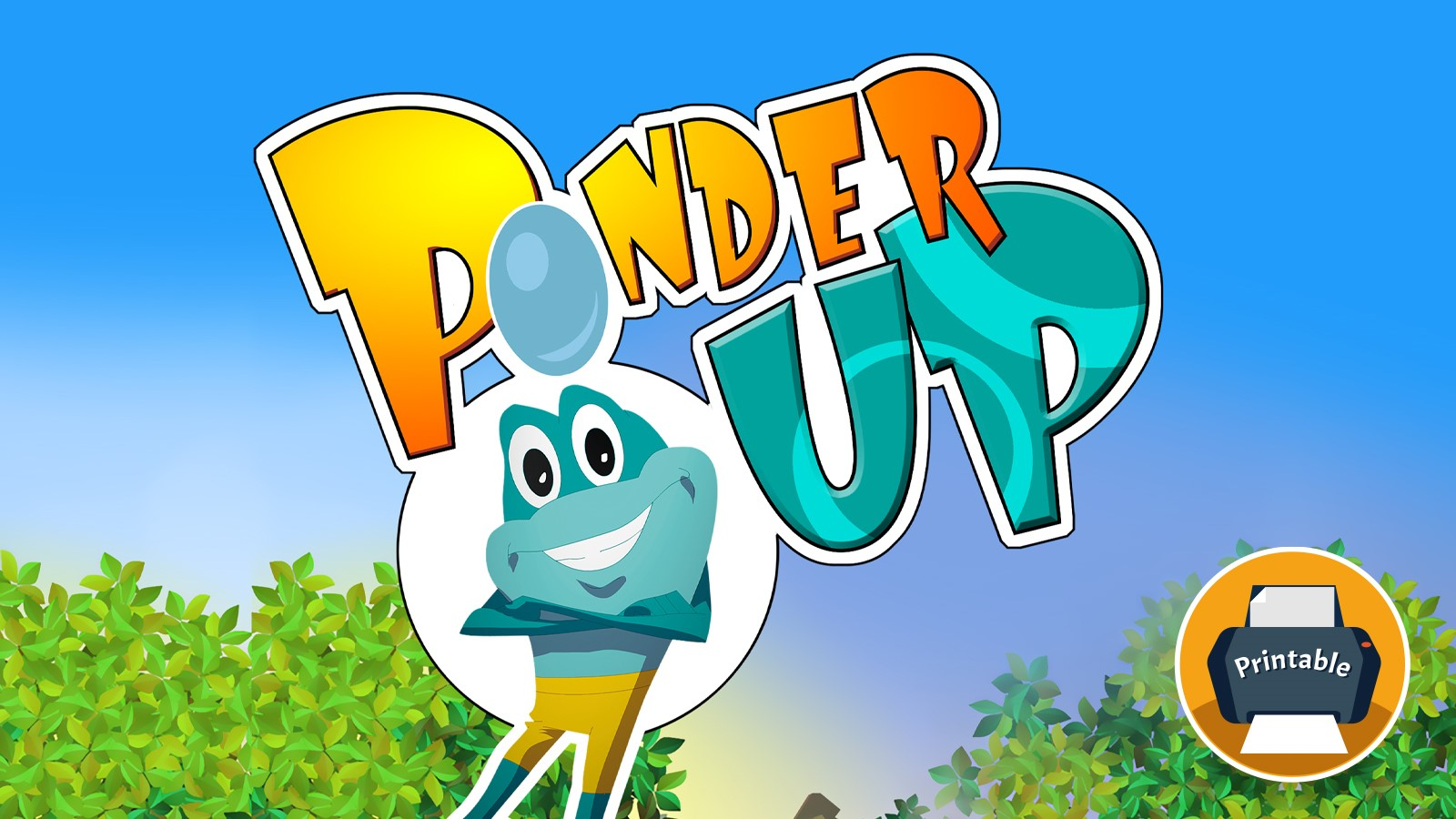Developing math skills is an ongoing process for all young students. Therefore, it shouldn’t stop when they are not in the classroom.

Ponder Up Board Game will help your children practice comparisons of numbers and quantities in a fun and engaging way in the convenience of their home with their family.

Welcome to the math pond where the little frog character helps you choose the correct answer for each question. There are two bubbles at the top of the page that contain numbers and/or quantities and/or shapes and/or math operations that need to be compared.

#### Game 1: Greater and Lesser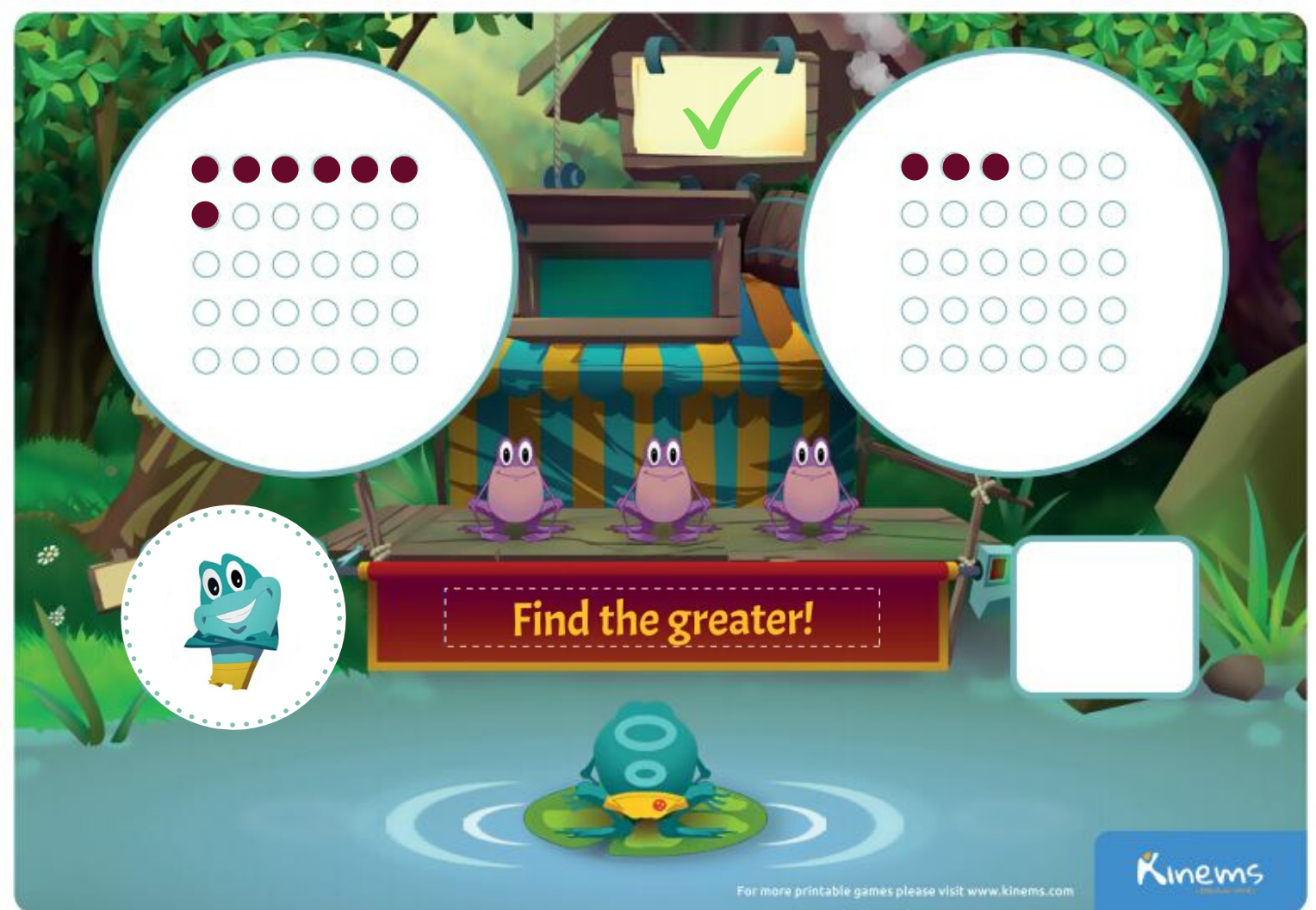Which is more? Use the game board on page 4 and dots that you cut out to go on top of the dots on the image.

For young children, keep it simple with numbers up to 5. For example, place three dots in the left circle and 1 in the right circle. Place the instructions “find the greater” in the space under the three frogs. Then, ask them to place the frog on the right answer.

If you cut the numerals from Math Operations, you have the greater sign cut out which you can have your child place in the green board between the two circles once they figure out which is the greater number or quantity. You can place the correct numeral under each of the circles.

Continue working with greater than and the sign with other number combinations. Turn the greater than sign pointing to the lesser and demonstrate how this works when the number on the left is less than the number or amount on the right.

#### Game 2: Odd or Even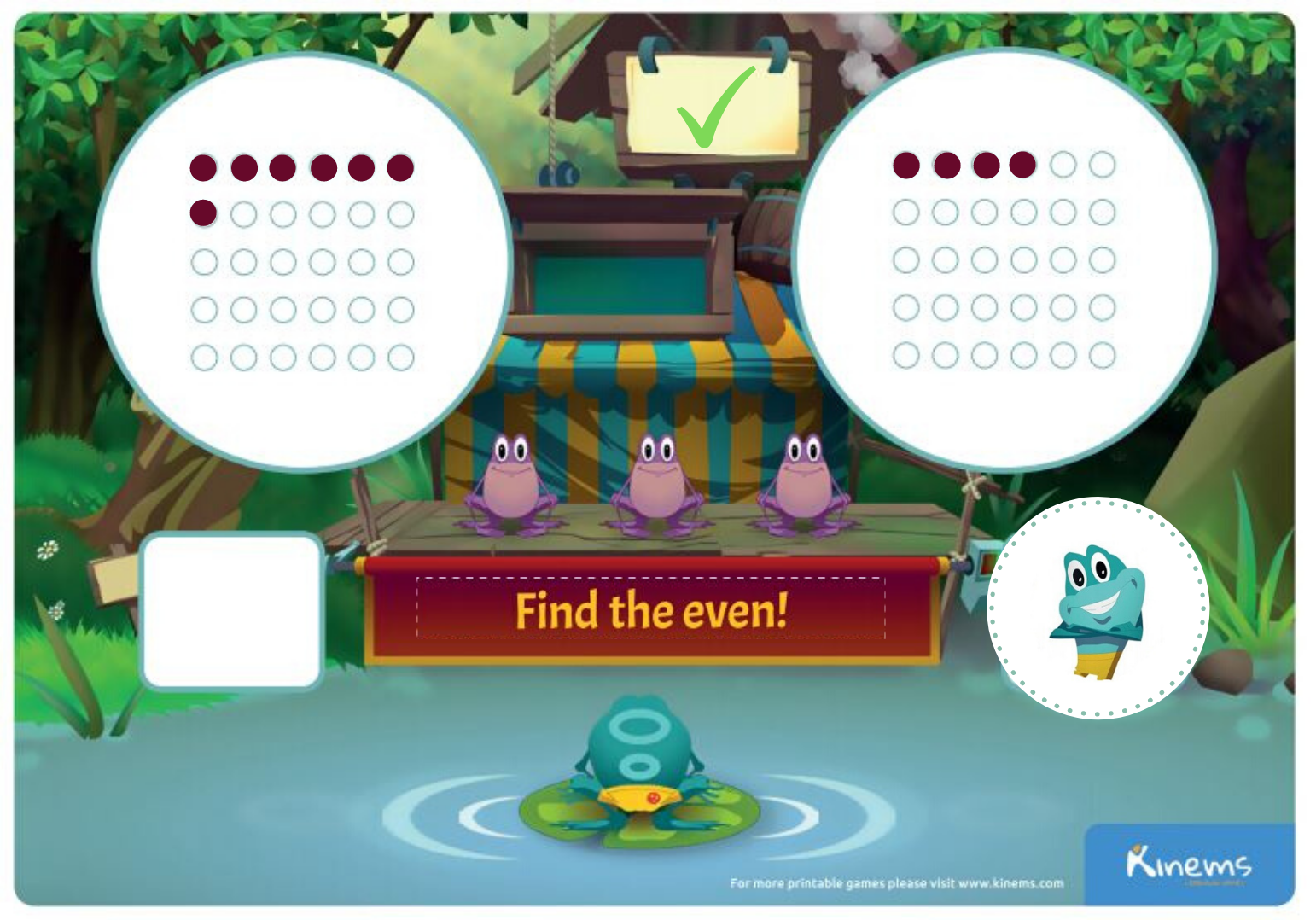In this game, you place dots or numerals in the circles. Make one circle an odd number and one circle an even number. Take the instructions “Find the Even” or “Find the Odd” and place it under the three frogs. Then ask your kids to place the frog on the correct answer.

#### Game 3: Ten more than the number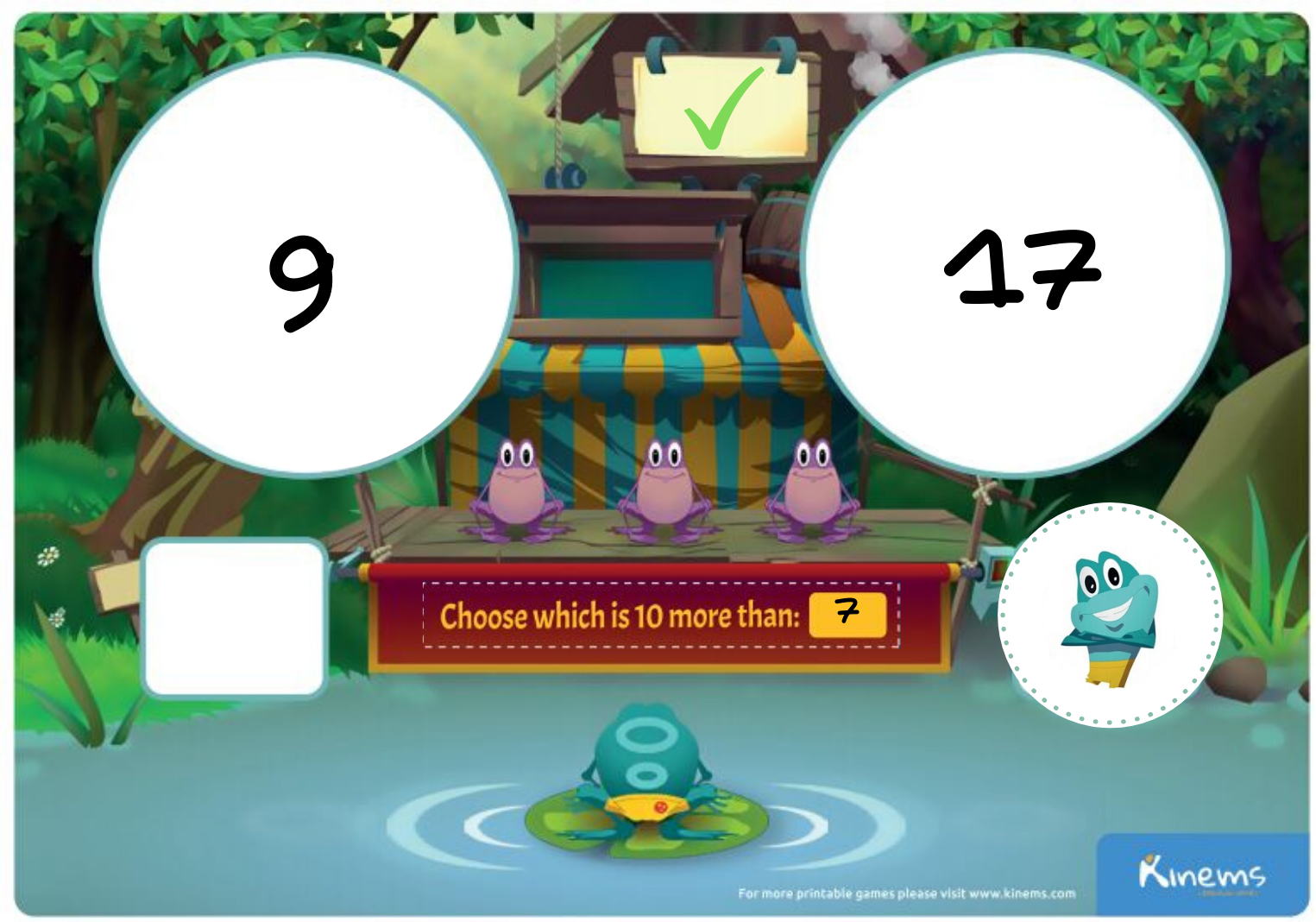This is good for first graders. Place one number in a circle and then place a number that has 10 more in the other circle. Start with teens for kindergarten and first graders. So for example, one circle could hold 7 and the other 17. Choose the direction that says: “Choose which is 10 more than _” and place it under the three frogs. Have your child put a frog on the correct number.

#### Game 4: One hundred more than the number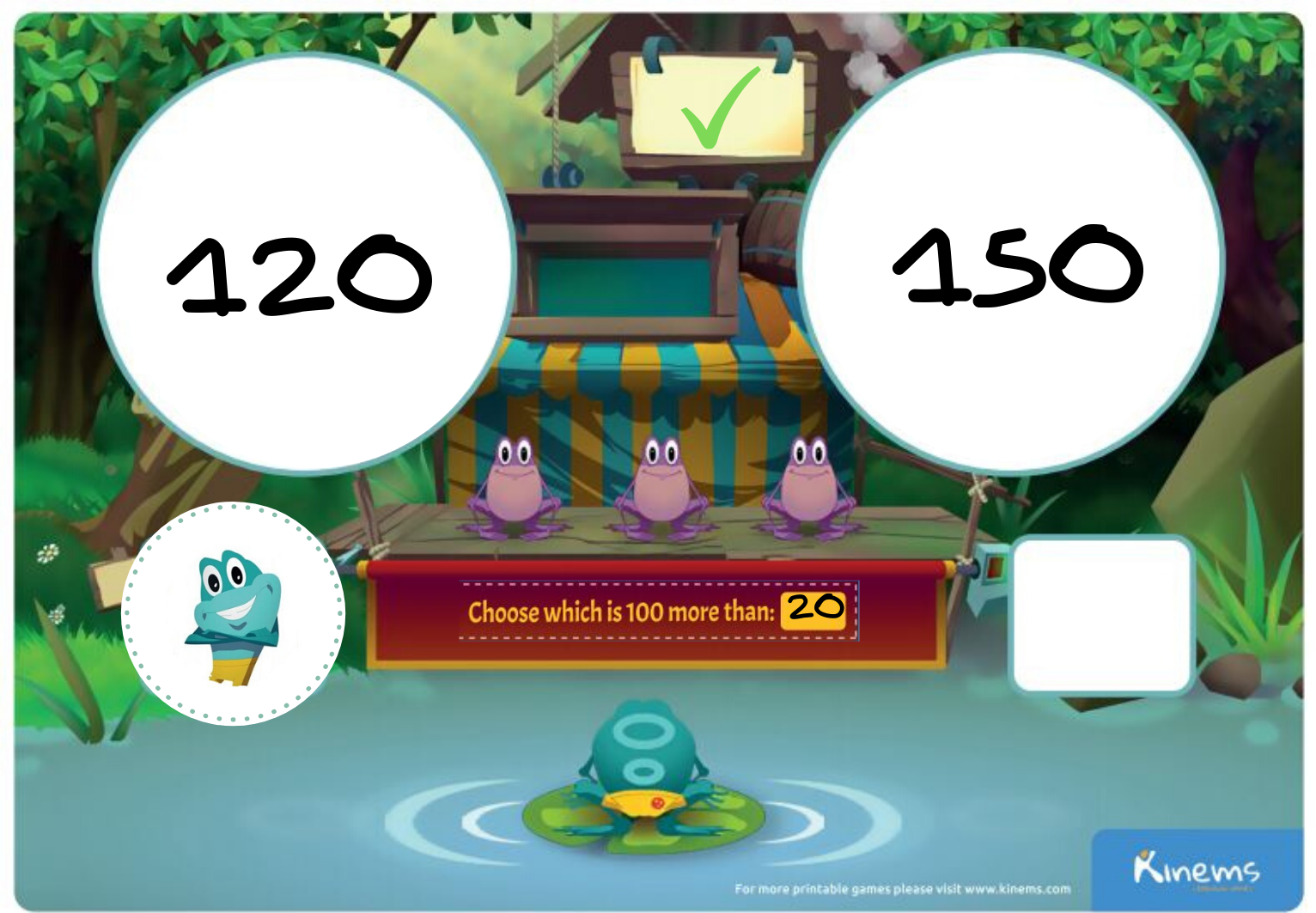Use this game with second graders. Place a number that is 100 more than a number you chose and place each in the circles. Add the instruction: “Choose which is 100 more than __”
Have your child come up with the same problems for you.

#### Game 5: Shapes and angles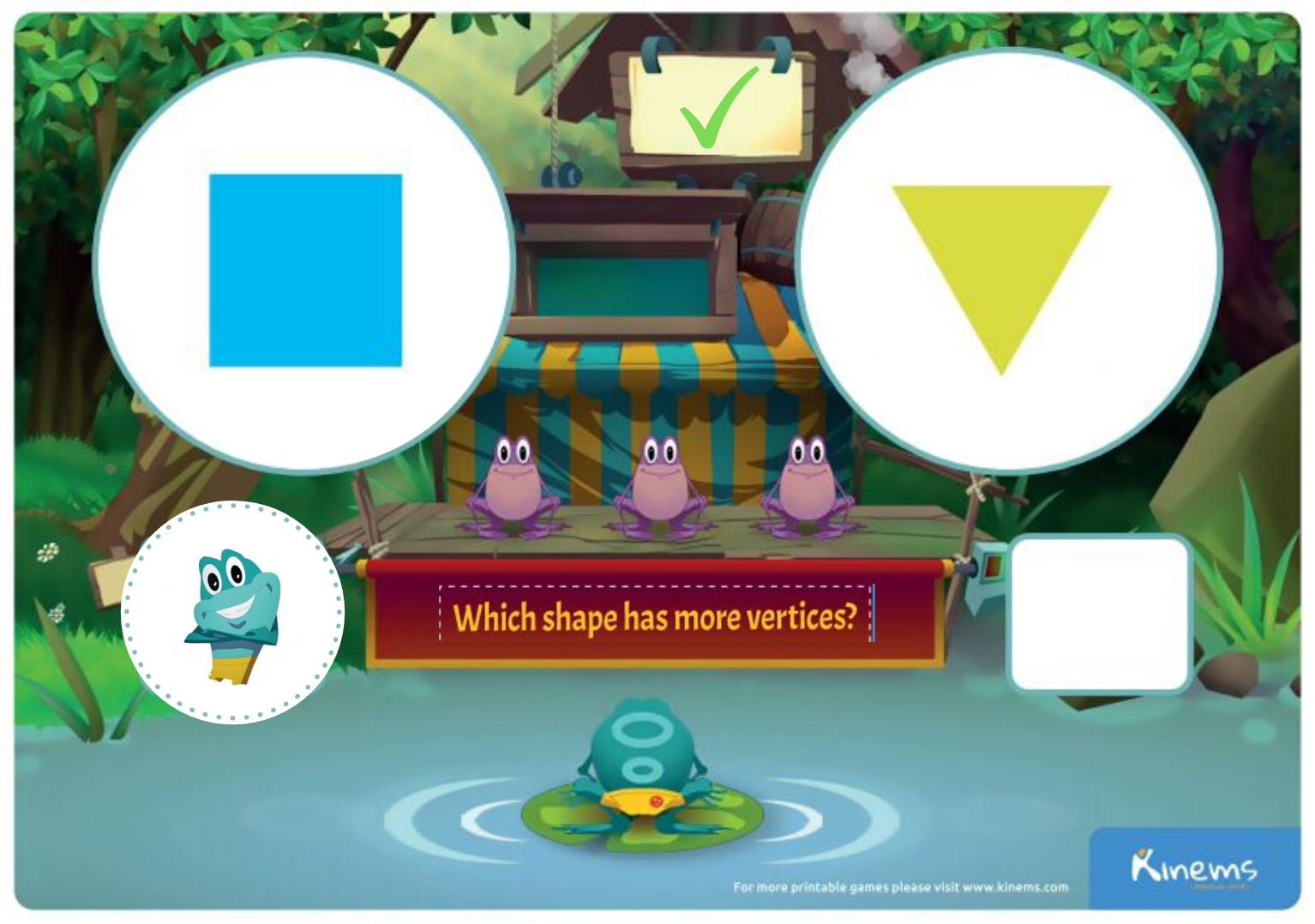Using the cut out shapes, choose two to compare that have more angles or vertices or “corners”. You can choose a triangle and a square. Or a triangle and a pentagon. (Make sure your child understands what an angle is before you quiz them.) Use the “Which Shape has more vertices” instruction to place under the three frogs. Then give them the frog to place on the right answer.

#### Here are the various goals for the Ponder Up game by grade level:

Pre-kindergarten Math

Identify whether the number of objects in one group is more, less, greater than, fewer, and/or equal to the number of objects in another group, e.g., by using matching and counting strategies (up to 5 objects).

Kindergarten Math

• Identify whether the number of objects in one group is greater than, less than, or equal to the number of objects in another group, e.g., by using matching and counting strategies.
• Compare two numbers between 1 and 10 presented as written numerals.
• Compare numbers. Identify whether the number of objects in one group is greater than, less than, or equal to the number of objects in another group, e.g., by using matching and counting strategies. (Include groups with up to ten objects.) Compare two sets and identify the set that is less than the other set, up to 10.
• Identify whether the number of objects in one group is greater than, less than, or equal to the number of objects in another group, e.g., by using matching and counting strategies.
• Identify the smaller or larger number given two numbers between 0 and 10
• Identify and describe shapes.
• Describe objects in the environment using names of shapes, and describe the relative positions of these objects using terms such as above, below, beside, in front of, behind, and next to.
• Correctly name shapes regardless of their orientations or overall size.
• Analyze, compare, create, and compose shapes.
• Analyze and compare two- and three-dimensional shapes, in different sizes and orientations, using informal language to describe their similarities, differences, parts (e.g., number of sides and vertices/"corners") and other attributes (e.g., having sides of equal length).
• Identify a group of fewer than 10 objects as odd or even.

• Compare two two-digit numbers based on meanings of the tens and ones digits, recording the results of comparisons with the symbols >, =, and <.
• Understand place value. Compare two two-digit numbers based on meanings of the tens and ones digits, recording the results of comparisons with the symbols >, =, and <.
• Given a two-digit number, mentally find 10 more or 10 less than the number, without having to count; explain the reasoning used.
• Using base ten blocks, find 10 more or 10 less of a given two-digit number (e.g., what is 10 more than 20? What is 10 less than 30?)
• Compare two-digit numbers up to 31 using representations and numbers (e.g., identify more 10s, less 10s, more 1s, fewer 1s, larger number, smaller number)
• Use place value understanding and properties of operations to add and subtract. Given a two-digit number, mentally find 10 more or 10 less than the number, without having to count; explain the reasoning used.

• Determine whether a group of objects (up to 20) has an odd or even number of members, e.g., by pairing objects or counting them by 2s; write an equation to express an even number as a sum of two equal addends.
• Compare two three-digit numbers based on meanings of the hundreds, tens, and ones digits, using >, =, and < symbols to record the results of comparisons.
• Reason with shapes and their attributes. Recognize and draw shapes having specified attributes, such as a given number of angles or a given number of equal faces. Identify triangles, quadrilaterals, pentagons, hexagons, and cubes. (Sizes are compared directly or visually, not compared by measuring.)
• Analyze and compare two- and three-dimensional shapes, in different sizes and orientations, using informal language to describe their similarities, differences, parts (e.g., number of sides and vertices/"corners") and other attributes (e.g., having sides of equal length).
• Use place value understanding and properties of operations to add and subtract.
• Mentally add 10 or 100 to a given number 100-900, and mentally subtract 10 or 100 from a given number 100-900. (e.g., What is 100 more than 500? What is 100 fewer than 700?)
• Mentally add or subtract 10 from a given set from the tens family (e.g., What is 10 more than 50? What is 10 fewer than70?)
• Work with equal groups of objects to gain foundations for multiplication. Determine whether a group of objects (up to 20) has an odd or even number of members, e.g., by pairing objects or counting them by 2s; write an equation to express an even number as a sum of two equal addends.

• Read and write multi-digit whole numbers using base-ten numerals, number names, and expanded form. Compare two multi-digit numbers based on meanings of the digits in each place, using >, =, and < symbols to record the results of comparisons.
• Understand place value. Compare two three-digit numbers based on meanings of the hundreds, tens, and ones digits, using >, =, and < symbols to record the results of comparisons.
• Compare three-digit numbers using representations and numbers (e.g., identify more hundreds, less hundreds, more tens, less tens, more ones, less ones, larger number, smaller number)
• Generalize place value understanding for multi-digit whole numbers. Read and write multi-digit whole numbers using base-ten numerals, number names, and expanded form. Compare two multi-digit numbers based on meanings of the digits in each place, using >, =, and < symbols to record the results of comparisons. (Grade 4 expectations in this domain are limited to whole numbers less than or equal to 1,000,000.)

Follow up activities to do at home:

• Arrange objects in even numbers. Sorting laundry socks is a great fun way to count by twos. Make songs out of counting by 2s and counting by 3s. Count by 5s and count by 10s.
• Go on a shape hunt in the house! How many rectangles can you find? Each window that has panes can be counted. Every drawer and cabinet can count. Make it into a game where family members predict how many rectangles there are in the house.
• Check out the pantry. Are there circles where there are cans? Are there rectangles where there are cereal boxes? Are there triangles in the tortilla chips? Are there circles in the cookies? Make tallies of what you find. You can then make a graph comparing the amount of each shape in the house.
• Use the symbols ” >, =, and <” to make real world comparisons. So when you lay out cereal or goldfish, make “equations” with real world objects and use the symbols.
• Get a clean jar and fill it with beans or goldfish or cereal. Then have your child record guesses from all family members as to how many are inside. You can get in touch with extended family like grandparents on a videocall and include them in the contest! When everyone has their guesses recorded, open the jar and have your child arrange the contents in piles of ten. Then count by tens to see how many are there and add in the ones. You can have them combine the tens into hundreds piles. If you have a good jar you might have a couple hundred of the contents and it would be a really fun learning experience with real world manipulatives.
• Go outdoors and find branches to compare. Count the leaves on each and see which is greater and which is lesser. If you have a fence, count the fence posts. Compare sides and see which is lesser/greater.
• If you live in a neighborhood with stoops, compare the number of steps in each stoop. Make a graph or map of the block and make greater/lesser comparisons.
• Count toys or possessions to make comparisons. Compare the number of magic markers to the number of crayons, etc.
• For young children, take a favorite stuffed animal or character and hide them under, inside and on top of things. When your child locates the object, they must say what position the object was using words like “under, inside, on top of, next to, etc.
• Play a game where you tell your young child to show you “under” by positioning themselves as under something. Use all of the words in a game like format. Have your child tell you or other family members the position to demonstrate.